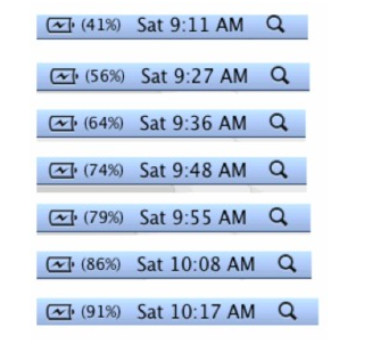# Bivariate Data

## Objective

Write equations to represent lines fit to data and make predictions based on the line.

## Common Core Standards

### Core Standards

?

• 8.SP.A.3 — Use the equation of a linear model to solve problems in the context of bivariate measurement data, interpreting the slope and intercept. For example, in a linear model for a biology experiment, interpret a slope of 1.5 cm/hr as meaning that an additional hour of sunlight each day is associated with an additional 1.5 cm in mature plant height.

?

• 8.F.B.4

## Criteria for Success

?

1. Write an equation in slope-intercept form for a line that represents a trend in data in a scatter plot.
2. Use the equation of a line of best fit to make predictions about new data.
3. Understand that predictions based on equations of best fit lines are based on the association between the data and do not represent actual data points.

## Tips for Teachers

?

Students recall and are re-engaged in their work with linear equations, specifically writing linear equations given a graph or information about the relationship.

#### Fishtank Plus

• Problem Set
• Student Handout Editor
• Vocabulary Package

## Anchor Problems

?

### Problem 1

The scatter plot below represents the relationship between a crocodile’s bite force (in pounds) and its body mass (in pounds) for several species of crocodiles. Patti drew a line through the graph to represent the trend in the data points.1. Write an equation to represent the line of best fit that Patti drew for the data.
2. Using your equation, predict the bite force of a 600-pound crocodile.

#### References

EngageNY Mathematics Grade 8 Mathematics > Module 6 > Topic C > Lesson 9Exercise 5

Grade 8 Mathematics > Module 6 > Topic C > Lesson 9 of the New York State Common Core Mathematics Curriculum from EngageNY and Great Minds. © 2015 Great Minds. Licensed by EngageNY of the New York State Education Department under the CC BY-NC-SA 3.0 US license. Accessed Dec. 2, 2016, 5:15 p.m..

Modified by The Match Foundation, Inc.

### Problem 2

Jerry forgot to plug in his laptop before he went to bed. He wants to take the laptop to his friend’s house with a full battery. The pictures below show screenshots of the battery charge indicator after he plugs in the computer at 9:11 a.m.1. The screenshots suggest an association between two variables. What are the two variables in this situation?
2. Make a scatter plot of the data.
3. Draw a line that fits the data and find the equation of the line.
4. When can Jerry expect to have a fully charged battery?

#### References

Illustrative Mathematics Laptop Battery Charge

Laptop Battery Charge, accessed on March 15, 2017, 12:45 p.m., is licensed by Illustrative Mathematics under either the CC BY 4.0 or CC BY-NC-SA 4.0. For further information, contact Illustrative Mathematics.

## Problem Set

?The following resources include problems and activities aligned to the objective of the lesson that can be used to create your own problem set.

• Include review problems for 8.F.4 where students are given graphs or tables and write the equation of the line.
• Revisit the problems from Lesson 4 where students drew lines to data sets. Have students write the equations for their lines of fit.

?

The scatter plot below shows the height and speed of some of the world’s fastest roller coasters. Use the scatter plot to answer the questions that follow.1. Draw a line through the data points that best represent the trend in the data.
2. Find the equation of your line.
3. A roller coaster has a height of 360 feet. What prediction can you make about its speed?

#### References

EngageNY Mathematics Grade 8 Mathematics > Module 6 > Topic C > Lesson 9Exit Ticket

Grade 8 Mathematics > Module 6 > Topic C > Lesson 9 of the New York State Common Core Mathematics Curriculum from EngageNY and Great Minds. © 2015 Great Minds. Licensed by EngageNY of the New York State Education Department under the CC BY-NC-SA 3.0 US license. Accessed Dec. 2, 2016, 5:15 p.m..

Modified by The Match Foundation, Inc.

?